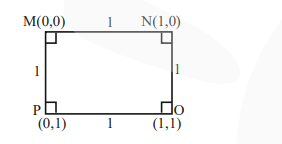# Solve this following

Question:

For the four circles $\mathrm{M}, \mathrm{N}, \mathrm{O}$ and $\mathrm{P}$, following four equations are given :

Circle $\mathrm{M}: \mathrm{x}^{2}+\mathrm{y}^{2}=1$

Circle $\mathrm{N}: \mathrm{x}^{2}+\mathrm{y}^{2}-2 \mathrm{x}=0$

Circle $\mathrm{O}: \mathrm{x}^{2}+\mathrm{y}^{2}-2 \mathrm{x}-2 \mathrm{y}+1=0$

Circle $P: x^{2}+y^{2}-2 y=0$

If the centre of circle $\mathrm{M}$ is joined with centre of the circle N, further centre of circle $\mathrm{N}$ is joined with centre of the circle $\mathrm{O}$, centre of circle $\mathrm{O}$ is joined with the centre of circle $P$ and lastly, centre of circle $P$ is joined with centre of circle $M$, then these lines form the sides of a :

1. Rhombus

2. Square

3. Rectangle

4. Parallelogram

Correct Option: , 2

Solution:

$M: x^{2}+y^{2}=1$    $(0,0)$

$N: x^{2}+y^{2}-2 x=0$  $(1,0)$

$O: x^{2}+y^{2}-2 x-2 y+1=0$   (1,1)

$P: x^{2}+y^{2}-2 y=0 \quad(0,1)$